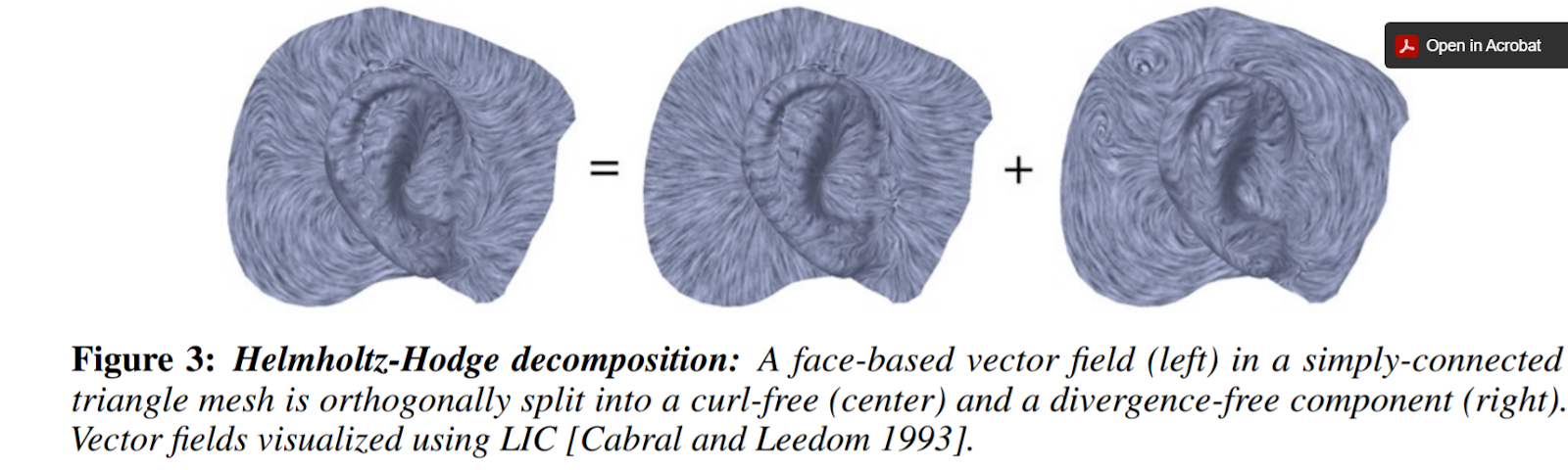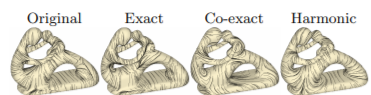Categories

## Understanding Vector Fields More Than In My Calculus 3 Class

AKA: Takeaways from Weeks 1-2 of High-order Directional Field Design Research

Author: Bryce Van Ross

It’s incredible how much one can learn in a month, and I’m looking forward to learning more (especially theoretically). In that same spirit, I highlight some takeaways from research made in my first SGI project. This project was guided by research mentor Dr. Amir Vaxman and TA Klara Mundilova, where I worked with fellow SGI student Jonathan Mousley.

Question(s): Usually we think of vectors and computations like divergence and curl as interrelated, and they are. But can we determine something more nuanced about these properties with respect to some vector field if we encounter a complex (i.e. having multiple vertices/edges) triangular mesh? Yes, we can. But it depends on your choice of approach of partitioning your mesh. For the sake of my research, we focus on the face-based representation.

Face-based representations of vector fields can then be broken down into vertex-based and edge-based approaches, per face per triangle. This means we are working with vector fields on faces that are gradients of (piecewise linear) functions that are either defined on the vertices or on the midpoints of edges. Depending on the choice of approach, then your computations are different. But which way is better and what are the consequences?

Answer(s): This is a natural question. Vertex-based (for certain reasons) seems to yield better approximations, which lead to better attempts at mimicry of continuity. In this sense, vertex-based computations are considered conforming, whereas edge-based computations are deemed nonconforming (w.r.t. continuity). Suppose we wanted to express a given vector field u in terms of familiar computations. Naturally, we would prefer to use vertex-based computations. However, we must remember that degrees of freedom (D.O.F.) must be maintained. Surprisingly, using purely vertex-based computations (or, purely edge-based computations) are in violation of D.O.F. More surprisingly, we find that our only solution is to use a mix of both the conforming and nonconforming terms. So, even though the gradients are distinct, both are equally valuable in terms of a reduction of u. So, there is a need to incorporate both $$G_v$$ (the vertex-based gradient) and $$G_e$$ (the edge-based gradient). This mixture could be complicated, but isn’t…it only requires the sum of 3 terms. The first term includes $$G_v$$ and computes the divergence but is curl-free. For the second term, including $$G_e$$, it computes the curl yet is div-free. The last term, referred to as $$h$$, is both divergence-free and curl-free. Note: that $$G_v$$ and $$G_e$$ can be interchanged w.r.t. the first two terms if such equation is multiplied by the rotation matrix $$J$$. Ultimately, u (or any vector space) has non-trivial representation (a.k.a. there’s more than meets the eye).

There’s more to it, but the above refers to the Helmholtz-Hodge Decomposition: $u = G_v\cdot f + J\cdot G_e\cdot g + h.$ A better visualization can be found below (Source: Vector Field Processing on Triangle Meshes), where the h term is not illustrated, in the topmost picture below. In the secondary picture, all components are expressed (Source: Subdivision Directional Fields, Figure 9, Top Row).